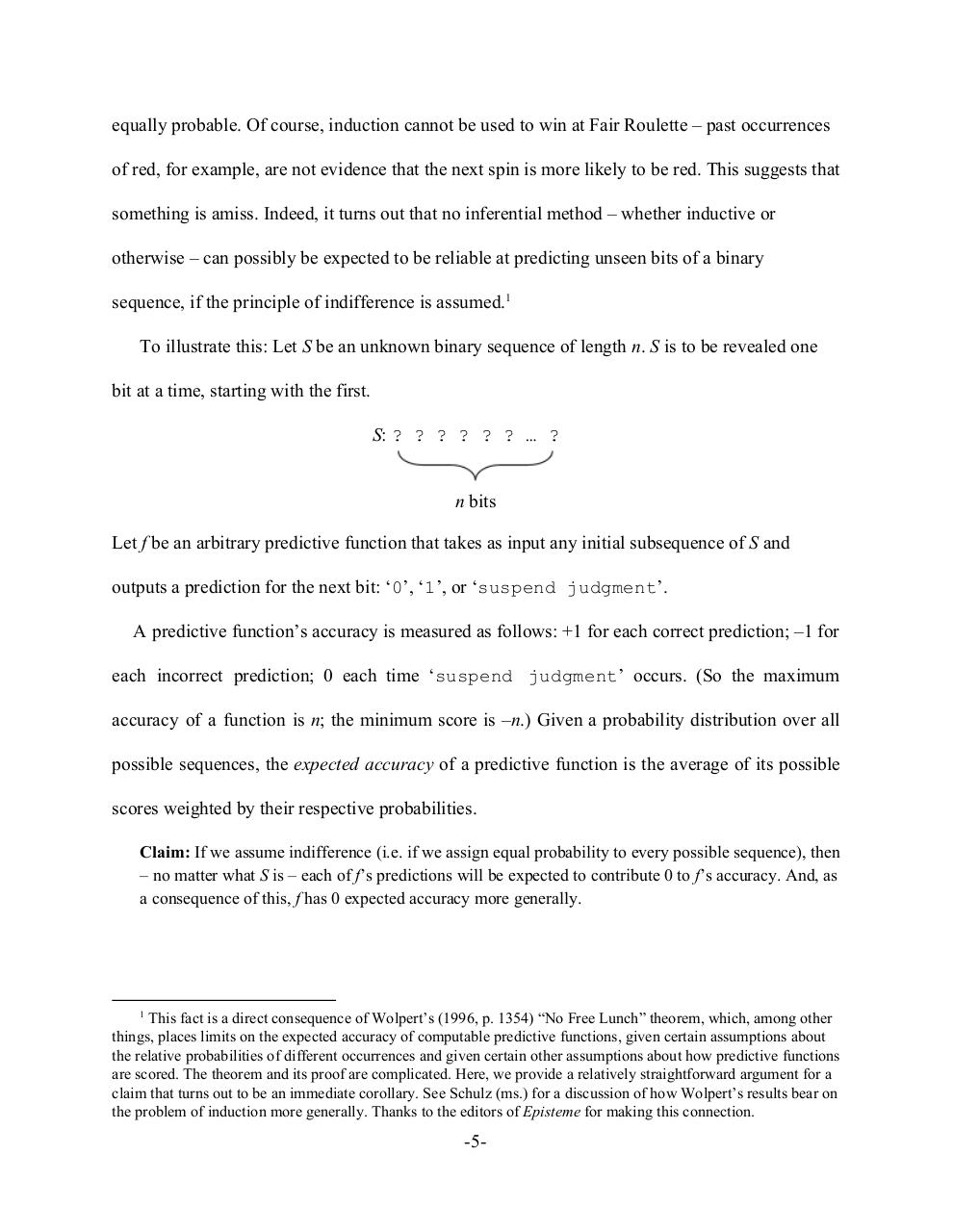PDF Archive

Easily share your PDF documents with your contacts, on the Web and Social Networks.

FoolMeOnce.pdfPage 1 2 3 4 5 6 7 8 9 10 11

Text preview

equally probable. Of course, induction cannot be used to win at Fair Roulette – past occurrences
of red, for example, are not evidence that the next spin is more likely to be red. This suggests that
something is amiss. Indeed, it turns out that no inferential method – whether inductive or
otherwise – can possibly be expected to be reliable at predicting unseen bits of a binary
sequence, if the principle of indifference is assumed.1
To illustrate this: Let ​S be an unknown binary sequence of length ​n. ​S is to be revealed one
bit at a time, starting with the first.
S: ​? ? ? ? ? ? … ?​ ​:​S
n bits
Let ​f be an arbitrary predictive function that takes as input any initial subsequence of ​S and
outputs a prediction for the next bit: ‘​0​’, ‘​1​’, or ‘​suspend judgment​’.
A predictive function’s accuracy is measured as follows: +1 for each correct prediction; –1 for
each incorrect prediction; 0 each time ‘​suspend judgment​’ occurs. (So the maximum
accuracy of a function is ​n; the minimum score is –​n.) Given a probability distribution over all
possible sequences, the ​expected accuracy of a predictive function is the average of its possible
scores weighted by their respective probabilities.
Claim: ​If we assume indifference (i.e. if we assign equal probability to every possible sequence), then
– no matter what ​S is – each of​ f’s predictions​ will be expected to contribute 0 to ​f’s accuracy. And, as
a consequence of this, ​f has 0 expected accuracy more generally.

1

This fact is a direct consequence of Wolpert’s (1996, p. 1354) “No Free Lunch” theorem, which, among other
things, places limits on the expected accuracy of computable predictive functions, given certain assumptions about
the relative probabilities of different occurrences and given certain other assumptions about how predictive functions
are scored. The theorem and its proof are complicated. Here, we provide a relatively straightforward argument for a
claim that turns out to be an immediate corollary. See Schulz (ms.) for a discussion of how Wolpert’s results bear on
the problem of induction more generally. Thanks to the editors of ​Episteme for making this connection.

-5-